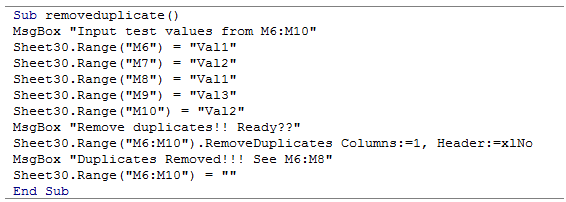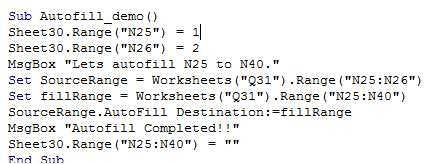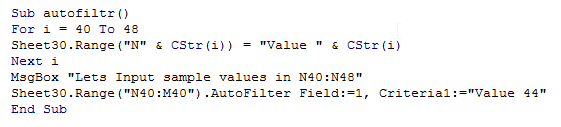## Vba advanced range methods in Excel

We had a brief introduction about Range Object and some of its methods and properties in the previous chapter.

Apart from the basic functionalities, there are some other methods which can be used to achieve advanced functions.

Some of the most often used functions are as discussed below.

RemoveDuplicates

This method is used to remove duplicate values from a range of values.The syntax for this method is as given below

The following example code illustrates this

1. Sub removeduplicate()
2. MsgBox "Input test values from M6:M10"
3. Sheet30.Range("M6") = "Val1"
4. Sheet30.Range("M7") = "Val2"
5. Sheet30.Range("M8") = "Val1"
6. Sheet30.Range("M9") = "Val3"
7. Sheet30.Range("M10") = "Val2"
10. MsgBox "Duplicates Removed!!! See M6:M8"
11. Sheet30.Range("M6:M10") = ""
12. End Sub

Autofill method

This method is used to perform autofill in a specified range.

This method might seem simple but without this, one has to go with For Loop which will make the program a little but slow

The following example illustrates this method

1. Sub Autofill_demo()
2. Sheet30.Range("N25") = 1
3. Sheet30.Range("N26") = 2
4. MsgBox "Lets autofill N25 to N40."
5. Set SourceRange = Worksheets("Q31").Range("N25:N26")
6. Set fillRange = Worksheets("Q31").Range("N25:N40")
7. SourceRange.AutoFill Destination:=fillRange
8. MsgBox "Autofill Completed!!"
9. Sheet30.Range("N25:N40") = ""
10. End Sub

Autofilter method

This method filters a list using Autofilter.This method takes multiple arguments.

The following example illustrates this

1. Sub autofiltr()
2. For i = 40 To 48
3. Sheet30.Range("N" & CStr(i)) = "Value " & CStr(i)
4. Next i
5. MsgBox "Lets Input sample values in N40:N48"
6. Sheet30.Range("N40:M40").AutoFilter Field:=1, Criteria1:="Value 44"
7. End Sub